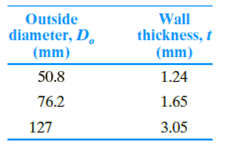Chapter 17, Problem 17.1PPrinciples of Geotechnical Enginee...

9th Edition
Braja M. Das + 1 other
ISBN: 9781305970939

Solutions

Chapter
SectionPrinciples of Geotechnical Enginee...

9th Edition
Braja M. Das + 1 other
ISBN: 9781305970939
Textbook Problem

ASTM D 1587-08 (2014) recommends the following dimensions for thin-walled steel tube sampling of soils for geotechnical purposes:Calculate the area ratio for each case and determine which sampler would be appropriate for the following soil characterization tests: grain size distribution, Atterberg limits, consolidation, and unconfined compression.

To determine

Find the area ratio for each case and appropriate sample for the given soil characterization tests.

The area ratio for tube 1 is 5.06%_.

The area ratio for tube 2 is 4.47%_.

The area ratio for tube 3 is 4.98%_.

Explanation

Given information:

The outside diameter (Do) for sample 1 is 50.8mm.

The outside diameter (Do) for sample 2 is 76.2mm.

The outside diameter (Do) for sample 3 is 127mm.

The wall thickness (t) for sample 1 is 1.24mm.

The wall thickness (t) for sample 2 is 1.65mm.

The wall thickness (t) for sample 3 is 3.05mm.

Calculation:

Calculate the inside diameter (Di) for tube 1 using the relation as follows:

(Di)=Dot (1)

Substitute 50.8mm for (Do) and 1.24mm for t in Equation (1).

(Di)=50.81.24=49.56mm

Find the area ratio for (Ar) tube 1 using the Equation as follows:

Ar(%)=Do2Di2Di2×100 (2)

Here, Di inside diameter of sample 1.

Substitute 50.8mm for Do and 49.56mm for Di in Equation (2).

Ar(%)=50.8249.56249.562×100=2,580.642,456.192,456.19×100=124.442,456.19×100=5.06%10%

Thus, the area ratio for tube 1 is 5.06%_.

Calculate the inside diameter (Di) for tube 2 using the relation as follows:

Substitute 76.2mm for (Do) and 1.65mm for t in Equation (1).

(Di)=76.21.65=74.55mm

Find the area ratio for (Ar) tube 2 using the Equation as follows:

Substitute 76.2mm for Do and 74.55mm for Di in Equation (2).

Ar(%)=76.2274.55274.552×100=5,806.445,557.75,557.7×100=248.745,557.7×100=4.47%10%

Thus, the area ratio for tube 2 is 4.47%_.

Calculate the inside diameter (Di) for tube 3 using the relation as follows:

Substitute 127mm for (Do) and 3.05mm for t in Equation (1).

(Di)=1273.05=123.95mm

Find the area ratio for (Ar) tube 3 using the Equation as follows:

Substitute 127mm for Do and 123.95mm for Di in Equation (2).

Ar(%)=1272123.952123.952×100=16,12915,363.6015,363.60×100=4.98%10%

Thus, the area ratio for tube 3 is 4.98%_.

A soil sample usually can be considered undisturbed if the area ratio is less than or equal to 10%.

• All the given three samples are considered as undisturbed sample, hence, it is suitable for consolidation and unconfined compression test.
• The Grain size distribution, atterberg limits are conducted only when the soil on remolded or disturbed soil, hence, sample disturbance is not a factor.

Thus, the all three samples are appropriate for all tests.

The Solution to Your Study Problems

Bartleby provides explanations to thousands of textbook problems written by our experts, many with advanced degrees!

Get Started

List four industries that depend on machining.

Precision Machining Technology (MindTap Course List)

What types of tip seals are used with cutting torch tips?

Welding: Principles and Applications (MindTap Course List)

Find all the answers to your study problems with bartleby.
Textbook solutions plus Q&A. Get As ASAP arrow_forward